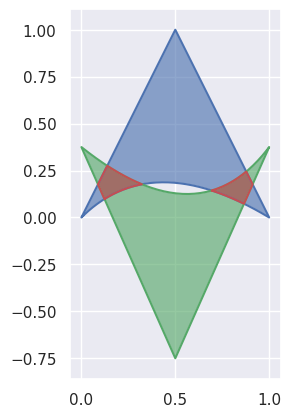# bezier¶

Helper for Bézier Curves, Triangles, and Higher Order Objects

This library provides:

Dive in and take a look!## Why Bézier?¶

A Bézier curve (and triangle, etc.) is a parametric curve that uses the Bernstein basis:

$b_{j, n} = \binom{n}{j} s^j (1 - s)^{n - j}$

to define a curve as a linear combination:

$B(s) = \sum_{j = 0}^n b_{j, n} \cdot v_j.$

This comes from the fact that the weights sum to one:

$b_{0, n} + b_{1, n} + \cdots + b_{n, n} = \left(s + (1 - s)\right)^n = 1.$

This can be generalized to higher order by considering three, four, etc. non-negative weights that sum to one (in the above we have the two non-negative weights $$s$$ and $$1 - s$$).

Due to their simple form, Bézier curves:

• can easily model geometric objects as parametric curves, triangles, etc.

• can be computed in an efficient and numerically stable way via de Casteljau’s algorithm

• can utilize convex optimization techniques for many algorithms (such as curve-curve intersection), since curves (and triangles, etc.) are convex combinations of the basis

Many applications – as well as the history of their development – are described in “The Bernstein polynomial basis: A centennial retrospective”, for example;

• aids physical analysis using finite element methods (FEM) on isogeometric models by using geometric shape functions called NURBS to represent data

• used in robust control of dynamic systems; utilizes convexity to create a hull of curves

## Installing¶

The bezier Python package can be installed with pip:

$python -m pip install --upgrade bezier$ python3.8 -m pip install --upgrade bezier
$# To install optional dependencies, e.g. SymPy$ python    -m pip install --upgrade bezier[full]


To install a pure Python version (i.e. with no binary extension):

\$ BEZIER_NO_EXTENSION=true \
>   python  -m pip install --upgrade bezier --no-binary=bezier


bezier is open-source, so you can alternatively grab the source code from GitHub and install from source.

## Getting Started¶

For example, to create a curve:

>>> nodes1 = np.asfortranarray([
...     [0.0, 0.5, 1.0],
...     [0.0, 1.0, 0.0],
... ])
>>> curve1 = bezier.Curve(nodes1, degree=2)


The intersection (points) between two curves can also be determined:

>>> nodes2 = np.asfortranarray([
...     [0.0, 0.25,  0.5, 0.75, 1.0],
...     [0.0, 2.0 , -2.0, 2.0 , 0.0],
... ])
>>> curve2 = bezier.Curve.from_nodes(nodes2)
>>> intersections = curve1.intersect(curve2)
>>> intersections
array([[0.31101776, 0.68898224, 0. , 1. ],
[0.31101776, 0.68898224, 0. , 1. ]])
>>> s_vals = np.asfortranarray(intersections[0, :])
>>> points = curve1.evaluate_multi(s_vals)
>>> points
array([[0.31101776, 0.68898224, 0. , 1. ],
[0.42857143, 0.42857143, 0. , 0. ]])


and then we can plot these curves (along with their intersections):

>>> import seaborn
>>> seaborn.set()
>>>
>>> ax = curve1.plot(num_pts=256)
>>> _ = curve2.plot(num_pts=256, ax=ax)
>>> lines = ax.plot(
...     points[0, :], points[1, :],
...     marker="o", linestyle="None", color="black")
>>> _ = ax.axis("scaled")
>>> _ = ax.set_xlim(-0.125, 1.125)
>>> _ = ax.set_ylim(-0.0625, 0.625)For API-level documentation, check out the Bézier Python package documentation.

## Development¶

To work on adding a feature or to run the functional tests, see the DEVELOPMENT doc for more information on how to get started.

## Citation¶

For publications that use bezier, there is a JOSS paper that can be cited. The following BibTeX entry can be used:

@article{Hermes2017,
doi = {10.21105/joss.00267},
url = {https://doi.org/10.21105%2Fjoss.00267},
year = {2017},
month = {Aug},
publisher = {The Open Journal},
volume = {2},
number = {16},
pages = {267},
author = {Danny Hermes},
title = {Helper for B{\'{e}}zier Curves, Triangles, and Higher Order Objects},
journal = {The Journal of Open Source Software}
}


A particular version of this library can be cited via a Zenodo DOI; see a full list by version.

bezier is made available under the Apache 2.0 License. For more details, see the LICENSE.